## Unity and Phi^3

Discussion concerning the first major re-evaluation of Dewey B. Larson's Reciprocal System of theory, updated to include counterspace (Etheric spaces), projective geometry, and the non-local aspects of time/space.
ckiit
Posts: 30
Joined: Sat Dec 21, 2019 7:54 am

### Re: Unity and Phi^3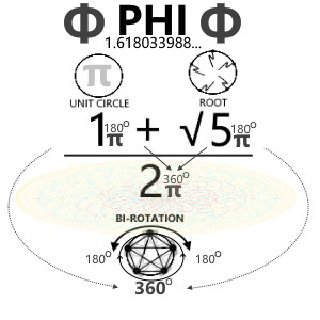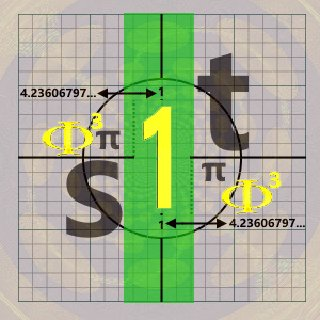As indicated by the first graphic, Φ can be understood as comprised of three components:
i. unit circle (typically 1+..., but π+ is recommended here, reasoning to follow)
ii. subjected to √5 (ie. five equidistant vertices about the circumference of i.)
iii. subjected to bi-rotation as 2π

Working from the back up, the bi-rotation is extremely important because it aligns with the important work of Prof. KVK Nehru:

https://reciprocalsystem.org/PDFa/On%20 ... Nehru).pdf
Conclusions

The Paper basically attempts at elucidating the nature of rotation in the context of the Reciprocal System, and correcting some likely misconceptions. Some of the important conclusions are summarized as follows:

1)It is emphasized that rotational motion is as primary as linear motion and that the simple harmonic motion (which is apparently an accelerated motion) inherent in photons is uniform birotation.
2)The inability of the conventional reference system to represent rotation completely and correctly results in a failure to distinguish between the inward and outward scalar directions of a rotational representation, and renders both the LF and the HF vibrations observable in the reference system.
3)The circular polarization of photons is the result of interaction with existing rotation/birotation in the medium and is accompanied by angular momentum.
____________________________________________________________________________________________________________________________________
1) Uniform birotation is consistent with the uniform dispersion resulting from 2π: simply (↔π↔π↔)
2) Failure to distinguish between the inward and outward scalar directions of a rotational representation has a certain critical metaphysical counter-part:
failure to distinguish between any one of any dichotomy for the other, such as true/false, right/wrong, good/evil etc. due to the same: failure to distinguish between scalar "directions"
3) 'Medium' is itself rooted in rotation/birotation.

Returning to Φ,
π+π√5
‾ 2π ‾
satisfies this basic format:
1) applies to 2π for there being a uniform birotation,
2) applies to √5 concerning which "direction"/"orientation" the pentagram is, and
3) applies to π instead of simply an arbitrary '1'

The latter is the reasoning for why
1+√5
‾‾ 2 ‾‾ = 1.618...
is not as inductively rooted as:
π+π√5
‾ 2π ‾ = 1.618...
which naturally produces the unit circle r = 1
if/when squared/reduced:
3π+π√5
‾ 2π ‾ = 2.618...
or simply Φ + 1, a difference of 2π.

Thus, understanding 1 as an expression of 2π ie. {one full rotation},
as Prof. Nehru similarly alludes to with the bi-rotational model of the photon,
lends itself to there being an internal intrinsic symmetry which obeys the "golden rule".

The following root form of Φ might give some insight as to how this symmetry operates internally: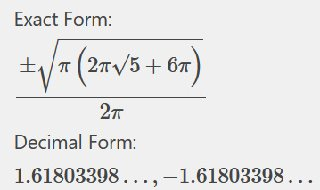______________________________________________
The root of π (one half-rotation) multiplied by {two "five-rooted" rotations,
(equivalent to one full rotation) plus three full rotations}
all over {one full rotation} equals the golden mean.

If RS(2) can focus in on this golden mean such to inductively root in it, the birotation model
describing the behavior of the photon itself (ie. light esp. as it may relate to consciousness itself)
will allow RS(2) will be in a position to posit an extremely potent 'theory of everything' that itself rests on Φ,
the potency being that birotation is 'found' intrinsic to Φ, thus would satisfy a true universal 'theory of everything'
to the same degree(s) to which Φ is manifestly present in the physical universe in which we live.

The outcome of this will reveal that the pentagram
(ie. Φ, as containing √5) has both:
intrinsic bi-rotation and bi-orientation,
thus can be used as a universal framework
to frame/resolve any/all particular displacements
concerning unity as c = 1.

user737
Posts: 74
Joined: Wed Oct 24, 2018 7:39 pm

### Re: Unity and Phi^3

davelook wrote:
Mon Mar 17, 2008 11:45 am

Not sure if this is relevant, but did you read those fascinating papers "The Euler Identity – a Radial Mathematical Interpretation 1 & 2"? I was getting ready to post on the amazing property of the "roots of unity"... the roots of unity are just the complex unit circle divided into e((2π)/x)i equal parts!

So the 2 square roots of unity are 1, -1. or e((2π)/2)i

The 3 cube roots are 1, (-.5+.866i), (-.5-.866i) or e((2π)/3)i

the 4 fourth roots are 1, -1, i, -i or e((2π)/4)i

the fifth roots form a pentagon in the unit circle, or e((2π)/5)i

....every 1/x power of 1 has a complex form as a x-agon in the unit circle!
Infinite Rider on the Big Dogma

ckiit
Posts: 30
Joined: Sat Dec 21, 2019 7:54 am

### Re: Unity and Phi^3

user737 wrote:
Wed Feb 12, 2020 3:41 pm
davelook wrote:
Mon Mar 17, 2008 11:45 am

Not sure if this is relevant, but did you read those fascinating papers "The Euler Identity – a Radial Mathematical Interpretation 1 & 2"? I was getting ready to post on the amazing property of the "roots of unity"... the roots of unity are just the complex unit circle divided into e((2π)/x)i equal parts!

So the 2 square roots of unity are 1, -1. or e((2π)/2)i

The 3 cube roots are 1, (-.5+.866i), (-.5-.866i) or e((2π)/3)i

the 4 fourth roots are 1, -1, i, -i or e((2π)/4)i

the fifth roots form a pentagon in the unit circle, or e((2π)/5)i

....every 1/x power of 1 has a complex form as a x-agon in the unit circle!
Thank you again - all certainly moving in the right direction.

Whatever this was concerning: extremely relevant. This is essentially confirming the relationship between 2π and 1,
the fifth root forming a pentagon in a circle being the definitive icing on the cake, as it relates directly back to √5 and thus Φ.
The 3 cube roots equaling -.5 and .866i are pointing to what I alluded to earlier concerning the RH: all real-number values must take the value of 1/2.

To tie all of this into the concept of CKIIT and birotation:

apex: capacity to choose one-way or the other (bi-direction, equal capacity for good/evil and/or right/wrong)
leg: to Know *{tree of living}
arm: All *{alpha}
arm: Not *{omega}
leg: to Believe *{tree of knowledge of good and evil}
__________________________________________
*{to Know all thus: not to Believe} tends towards any possible all-knowing 'state' (theist/atheist-invariant) concerning unity
*{to Believe all thus: not to Know} tends towards any possible all-belief-based 'state' which includes all ignorance(s), (dis)orientations and/or (dis)placements concerning unity
*CKIIT hypotheses

Internally, each orientation draws the related symmetrical bidirectional pentagram inside the unit circle.
Externally, the unit circle rotates/moves in/out accordingly concerning the two trees (CKIIT context) and/or unity (RS2 context).

If looking at the "believer vs. unbeliever" situation, in light of the two possible orientations,
the "believing" orientation points towards all possible belief-based ignorance(s) causing/sustaining suffering/death, whereas
the "knowing" orientation points towards all possible knowledge(s) ceasing suffering/death.

The only thing needed to capture these two orientations is precisely what Φ provides if/when generated with π:
π is one half rotation
+π√5 is another half rotation with five equidistant roots
‾‾2π‾‾ is the completed circle.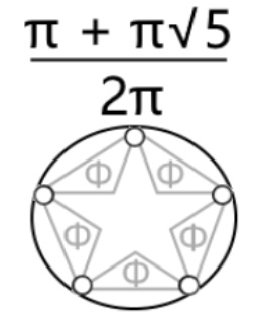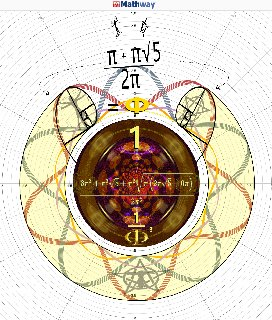user737
Posts: 74
Joined: Wed Oct 24, 2018 7:39 pm

### Re: Unity and Phi^3

A lot of synchronicity to other posts from davelook:
https://reciprocal.systems/phpBB3/viewt ... ?f=7&t=156
Infinite Rider on the Big Dogma

ckiit
Posts: 30
Joined: Sat Dec 21, 2019 7:54 am

### Re: Unity and Phi^3

user737 wrote:
Sun Feb 16, 2020 12:55 pm
A lot of synchronicity to other posts from davelook:
https://reciprocal.systems/phpBB3/viewt ... ?f=7&t=156
Regarding this, and:
bperet wrote:
Sat Feb 09, 2008 2:03 pm

Horace wrote:
And why is this remarkable ?

...because PI is the half of the natural counterspace unit (turn) ?
The turn in counterspace is unbounded; it has infinite angle so it would just be an integer count. Now, when you have to project that turn into observable space, there is no way to represent the concept of an unbounded/infinite angle, since you have to use the laws of the observable environment which say that a "rotational motion" is BOUNDED (like linear motion is bounded in counterspace as a vibration). So a Turn of 'n' angle becomes a rotation of n/2(2*PI) in space.

Looking at Dave's concepts and references, I see three connected factors:
1. They are spatial measurements of counterspatial events.
2. They are scale variant, requiring a series expansion to define ("hall of mirrors" measure).
3. The numerical coincidences are shadows on the wall of Plato's cave... projections.
One can learn a lot from shadows, but I think it would be far more interesting to find what is casting those shadows, then extract the "coincidences" from that projection. That would be pretty awesome.[/]
The turn in counterspace is indeed unbounded: magnitudes refer to arcs whose frequency is dictated by one full rotation, the variation being time. However, it is not so if/when coupled with Φ, which yields a real solution to a quadratic.
If/when the unbounded π is "bound" to/by Φ, the geometry that is intrinsic to the latter becomes intrinsic to π. This makes sense because π alone is transcendental, whereas Φ resolves as a quadratic.

Reading over the other posts, I collect the same Bruce did: this user is finding geometric relationships in numbers, as I see them as well.
I will endeavor to draw these relationships out more clearly over time, as they are certainly important. For example:

(π ± π√5)/2π = Φ, -0.618...
(π ± π√5)²/4π² = 2.61803398…(Φ + 1), 0.38196601…
2.61803398…+ 0.38196601…=2.99999999...=3
2.61803398…x 0.38196601…=0.99999999...=1
________________________________________
viz. how two decimals complete a whole via binding π to Φ
if/when subject to both: additive (+) and multiplicative (x) operations.

This digs at the root of the following:
bperet wrote:
Sun Apr 05, 2009 7:01 pm
Hey Dave...

I was working on some graphics regarding the Plane at Infinity and happen to notice that when the 3 orthogonal axes, X, Y and Z are projected onto the Plane at Infinity (or the Plane at Zero), they make points--one point for each axis, and one can draw a circle through those 3 points, where each point is separated by an arc 120° or 2π/3 radians--which look exactly like the 3rd roots of Unity that you have mentioned before, separating masses. Only difference being that the 3rd roots of unity would require a 3-axis projection with ONE real and TWO imaginary axes.

Could be that the masses are just the same motion, oriented on different coordinate time axes.
I suggest another possibility to the 3-axis projection: one real, one imaginary, one some particular configuration of both simultaneously generated/perceived by the observer. This concerns/addresses the observer principle:
bperet wrote:
Thu Aug 16, 2007 12:01 pm
davelook wrote:
But I have another question; why is 22.3505843 so darn close to sqrt5, 2.23607?
The sqrt(5) is the basis for the golden ratio, which shows up frequently when working with the coupling between the spatial and counterspatial reference frames, particularly in the biological realm. Dan loaned me some books on plants, showing the geometry of growth patterns being related by the phi ratio. Things like buds and leaf patterns can be calculated by creating linkage between space and counterspace, and plotted out with remarkable detail.

It may have something to do with the 'wavicle' (a discrete unit of counterspace) coupling to a 'particle'.

Just need to remember that we are dealing with the coordinate reference system in these cases, not the scalar motion, so the observer principle has to be accounted for...
Indeed √5 is the basis for the golden ratio, coupling between spacial and counterspacial relationships:
each 2π "arcs" concerns an equivalent "linear" pentagram (√5),
thus line and curve are reciprocal expressions of the same if/when "bound" by Φ²
whose internal construction sums exactly to '3' if adding both ± solutions together.

The arithmetic expression earlier derived for use with CKIIT adopts this idea:
produce a geometric expression which simultaneously equals '1' and some operation of π-based Φ.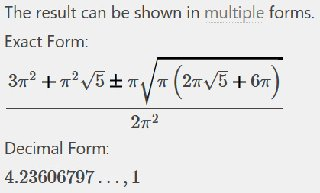In this case, Φ³ and 1 captures anything in a s³/t and/or t/s³ as s=1 and/or t=1
as 1/Φ^-3 equals the same as Φ³. Here is a visual representing the relation: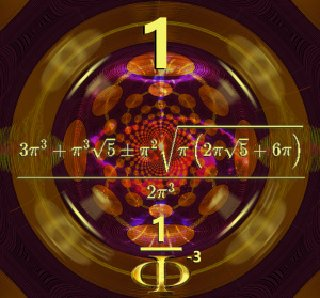Qualitatively, '9' becomes the fixed center-point,
(think two √5 pentagrams whose apexes are
one shared point, making 9 total points)
with relative 1-4 and 5-8 on either side,
(5/5)√5 - (1/5)√5 (for the concerned center point) leaves (4/5)√5 x 2 (birotation) = (8/5)√5,
4 "positive" and 4 "negative" ±(1-4) ±9 ±(5-8). This obviously
calls upon Tesla's allusion to the beauty of the 3/6/9 pattern:wherein (1, 2, 4)∞(8, 7, 5) captures/describes a folded circle (infinity) whose circumference is expressible as simply 2π,
each π containing four numerals, two of which concern 9 as their own triad 3-6-9
The real number system works exactly in this way, with -1/12 being the sum of all real numbers due to the -12/144
(qualitatively 1+2=-3 / 1+4+4=9) "barrier" relating to the above sum of (π ± π√5)²/4π itself equaling +3.

user737
Posts: 74
Joined: Wed Oct 24, 2018 7:39 pm

### Phi, the Divine Ratio

Infinite Rider on the Big Dogma

user737
Posts: 74
Joined: Wed Oct 24, 2018 7:39 pm

### Re: Unity and Phi^3

ckiit wrote:
Sun Feb 16, 2020 2:56 pm
(π ± π√5)/2π = Φ, -0.618...
(π ± π√5)²/4π = 2.61803398…(Φ + 1), 0.38196601…
First line works.
Second line is shown incorrectly (type-o as shown below in red).

Continuing thoughts:

Φ0 = 1

Φ1 = (π ± π√5) / 2π = 1.61803398… (Φ), -0.61803398…
1.61803398… + (-0.61803398…) = 1
1.61803398… × (-0.61803398…) = -1

Φ2 = ((π ± π√5)/2π)2 = (π ± π√5)2 / 4π2 = 2.61803398…(Φ + 1), 0.38196601…
2.61803398… + 0.38196601… = 3
2.61803398… × 0.38196601… = 1

What happens if we cube it.

Φ3 = ((π ± π√5)/2π)3 = (π ± π√5)3 / 8π3 = 4.23606797…(Φ2 + Φ), -0.23606797…
4.23606797… + (-0.23606797…) = 4
4.23606797… × (-0.23606797…) = -1

4th power:

Φ4 = ((π ± π√5)/2π)4 = (π ± π√5)4 / 16π4 = 6.85410196…(Φ3 + Φ2), 0.14589803…
6.85410196… + 0.14589803… = 7
6.85410196… × 0.14589803… = 1

5th power:

Φ5 = ((π ± π√5)/2π)5 = (π ± π√5)5 / 32π5 = 11.09016994(Φ4 + Φ3)…, -0.09016994…
11.09016994… + (-0.09016994)… = 11
11.09016994… × (-0.09016994)… = -1

Recovered pattern:
Sn+1 = Sn + Sn-1
Pn+1 = -Pn

Where S is sum and P is product.

First, add one, then multiply the quotient by Φ to create the series.

Also note that as n→∞, the ratio of Sn+1 / Sn → Φ

For example, sum of (±) solutions for Φ18 = 5778 & sum of (±) solutions for Φ19 = 9349

9349 / 5778 = 1.618033…

In other words, (Φn+1 + Φn) / Φ approaches 1 (or π) as n increases.
Infinite Rider on the Big Dogma

ckiit
Posts: 30
Joined: Sat Dec 21, 2019 7:54 am

### Re: Phi, the Divine Ratio

user737 wrote:
Sun Feb 16, 2020 4:43 pm
ckiit wrote:
Sun Feb 16, 2020 2:56 pm
(π ± π√5)/2π = Φ, -0.618...
(π ± π√5)²/4π = 2.61803398…(Φ + 1), 0.38196601…
First line works.
Second line is shown incorrectly (type-o as shown below in red).

Continuing thoughts:

...

Recovered pattern:
Sn+1 = Sn + Sn-1
Pn+1 = -Pn

Where S is sum and P is product.

First, add one, then multiply the quotient by Φ to create the series.

Also note that as n→∞, the ratio of Sn+1 / Sn → Φ

For example, sum of (±) solutions for Φ18 = 5778 & sum of (±) solutions for Φ19 = 9349

9349 / 5778 = 1.618033…

In other words, (Φn+1 + Φn) / Φ approaches 1 (or π) as n increases.
Sorry about the error - thanks for catching it.

I think there is something important to be said about a whole number having two particular decimal numbers who share some relationship to other whole numbers
regardless of the "order" of the operation (ie. addition, multiplication). I have a feeling this relates to the squaring (ie. multiplying something by itself) of Φ to produce itself + 1,
thereby creating a "terminal" for all numbers two "recede" back from a second-order operation (ie. multiplication) to a first one (ie. addition).

My idea is instead of using 1 and/or 1/2 units to depicts this addition of a "whole",
let π describe this as a "whole" 2π circle wherein two Φ operations (ie. spirals) interact to produce it.
user737 wrote:
Sun Feb 16, 2020 4:20 pm
Phi, the Divine Ratio by Bruce
Thank you - let's see what happens when we set each "1/2 unit" as π.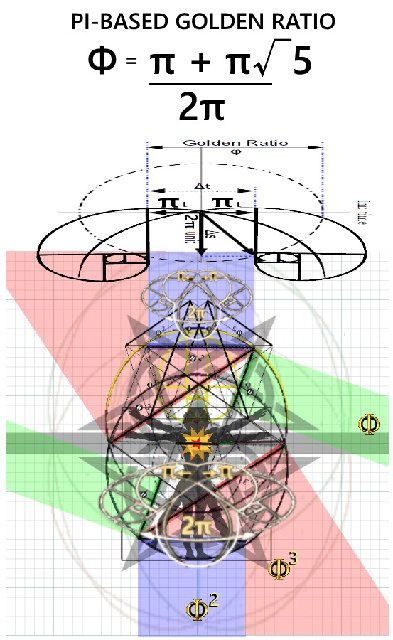Φ (green) makes twin-108º apexes whose adjoining base becomes the generated 72º (36ºx2) square of Φ,
Φ² (blue) as two equivalent half-circle Fibonacci spirals at 180º angles (2π) multiplied by the initial Φ gives
Φ³ (red) capable of producing any 3D geometry.

user737
Posts: 74
Joined: Wed Oct 24, 2018 7:39 pm

### Re: Phi, the Divine Ratio

ckiit wrote:
Tue Feb 18, 2020 6:47 am
I think there is something important to be said about a whole number having two particular decimal numbers who share some relationship to other whole numbers regardless of the "order" of the operation (ie. addition, multiplication). I have a feeling this relates to the squaring (ie. multiplying something by itself) of Φ to produce itself + 1, thereby creating a "terminal" for all numbers two "recede" back from a second-order operation (ie. multiplication) to a first one (ie. addition).
This is mathematical "geometric" dimensional reduction as noted in the earlier analysis of phi ("playing_with_phi.xlsx") and would be representative of the geometric-less "projective geometry" (PG) of scalar relationships (magnitude only, no direction) where geometric concepts have their corollary in mathematics. Before you can have geometry (PG→affine→metric/similar→Euclidean) you must first have displacements from unity.

The second order operation (square) is reduced to a first order operation (power of one). This is similar in nature as to how two bi-rotations combine to create a single linear harmonic displacement (i.e. a vibration). Just as one can become two; two can become one.

Combine two Euler formulas (of opposite rotation).
Note: "direction" is either outward (+) or inward (-)

exi + e-xi = 2cos(θ)

What required two dimensions (two 'x' values as above) reduce to a single variable (theta).
Two "imaginary" numbers (rotational operators) combine to create the REAL (i.e. temporal rotations share a spacial displacement).

Recall as well Euler's identity: e + 1 = 0... making i = √e or -1!

This is in keeping with earlier finding: the two (2) square roots of unity are 1, -1 or e((2π)/2)i where x is ±π!
ckiit wrote:
Tue Feb 18, 2020 6:47 am
let π describe this as a "whole" 2π circle wherein two Φ operations (ie. spirals) interact to produce it.
And let those two "half" spiral operations in Φ be in opposite aspects (positive versus negative)... would this not produce the circle?
ckiit wrote:
Tue Feb 18, 2020 6:47 am
Φ (green) makes twin-108º apexes whose adjoining base becomes the generated 72º (36ºx2) square of Φ,
Φ² (blue) as two equivalent half-circle Fibonacci spirals at 180º angles (2π) multiplied by the initial Φ gives
Φ³ (red) capable of producing any 3D geometry.
Have you seen this?:
https://archive.org/details/PythagorasP ... 3/mode/2up

What is the result should aspects of motion be inverted (i.e. time → space; space → time)?

Solve for conjugate relationship...

Can it be shown 1/Φ, 1/Φ-2, and 1/Φ-3 are similar result?
Infinite Rider on the Big Dogma

ckiit
Posts: 30
Joined: Sat Dec 21, 2019 7:54 am

### Re: Phi, the Divine Ratio

user737 wrote:
Tue Feb 18, 2020 7:46 am
This is mathematical "geometric" dimensional reduction as noted in the earlier analysis of phi ("playing_with_phi.xlsx") and would be representative of the geometric-less "projective geometry" (PG) of scalar relationships (magnitude only, no direction) where geometric concepts have their corollary in mathematics. Before you can have geometry (PG→affine→metric/similar→Euclidean) you must first have displacements from unity.

The second order operation (square) is reduced to a first order operation (power of one). This is similar in nature as to how two bi-rotations combine to create a single linear harmonic displacement (i.e. a vibration). Just as one can become two; two can become one.
This is certainly related to how I perceive the "believer vs. unbeliever" situation attempting to be solved for
as being both a local vibration (due to a being having roots in both: knowledge-negating-belief and belief-based ignorance)
and a 'state' vibration adjoining all "believers" whose vibration are rooted in the same shared 'state' belief.

If the most fundamental belief(s) are false, lacking knowledge of the same causes pain and suffering,
whereas having knowledge of the same would cease the same.
user737 wrote:
Tue Feb 18, 2020 7:46 am
Combine two Euler formulas (of opposite rotation).
Note: "direction" is either outward (+) or inward (-)

exi + e-xi = 2cos(θ)

What required two dimensions (two 'x' values as above) reduce to a single variable (theta).
Two "imaginary" numbers (rotational operators) combine to create the REAL (i.e. temporal rotations share a spacial displacement).

Recall as well Euler's identity: e + 1 = 0... making i = √e or -1!

This is in keeping with earlier finding: the two (2) square roots of unity are 1, -1 or e((2π)/2)i where x is ±π!
Very interesting - I see these two roots of unity as to/from unity ±c with the 2π as the "terrain".
Approaching unity would require alleviating all source(s) of displacement(s),
this obviously concerning belief-based ignorance(s) in the context of CKIIT.
user737 wrote:
Tue Feb 18, 2020 7:46 am
Have you seen this?:
https://archive.org/details/PythagorasP ... 3/mode/2up

What is the result should aspects of motion be inverted (i.e. time → space; space → time)?

Solve for conjugate relationship...
I have not seen the document, however have seen some of the author's YT content
and did draw from it. I am going to keep it open and continue to read as needed.

Concerning the questions, I do not know presently, as it must be obvious I am not a learned mathematician as
I drew the geometric relationships from Genesis 1:1 (vesica piscis) and realized Φ can draw this naturally only recently.

The following vesica piscis is constructed naturally by Φ thus inductively serves as a basis for much: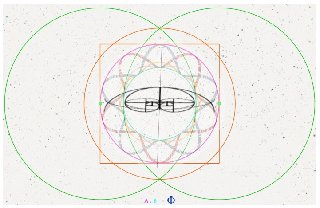including the CKIIT concept: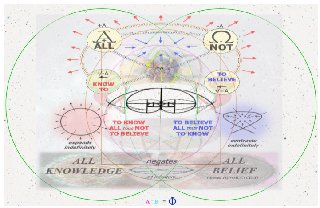Such a universal construct employs a simple quadropole
whose dipoles are denials of one another: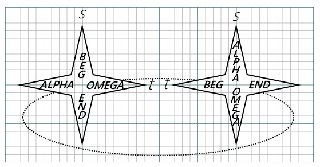The alpha∞omega is a null binary which satisfies all possible binaries: yes/no, 1/0, up/down etc.
The beg∞end is a null framework for any temporal (ie. displaced) phenomena: of suffering, for example.
By setting the latter to 'to know' and 'to believe' there is revealed the bi-rotation that moves to/from all-knowing.
In the very least: it clarifies what 'knowledge' is and/or serves: the negation of belief-based ignorance(s).

By treating this quadropole as the 2.23606... x 2.23606... = 5,
√5 can be seen as this quadrupole axis of 4 with 1 remaining for a navigator (ie. consciousness).
user737 wrote:
Tue Feb 18, 2020 7:46 am
Can it be shown 1/Φ, 1/Φ-2, and 1/Φ-3 are similar result?
I am sure it can be - I myself do not comprehend this in terms of quantitative math,
and will spend some time looking for the relationships. I have Euclid's Elements here
and should probably read it before going too much further with the math - it only needs
to serve the purpose of ceasing human suffering by clarifying a/the universal axis to/from unity.